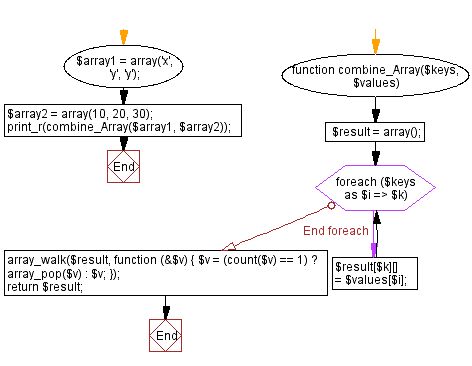﻿ PHP Array Exercise: Combine two arrays - w3resource# PHP Array Exercises : Combine two arrays

## PHP Array: Exercise-58 with Solution

Write a PHP script to combine (using one array for keys and another for its values) the following two arrays.

('x', 'y', 'y'), (10, 20, 30)

Sample Solution:

PHP Code:

``````<?php
function combine_Array(\$keys, \$values)
{
\$result = array();
foreach (\$keys as \$i => \$k) {
\$result[\$k][] = \$values[\$i];
}
array_walk(\$result, create_function('\$v', '\$v = (count(\$v) == 1)? array_pop(\$v): \$v;'));
return    \$result;
}
\$array1 = array('x', 'y', 'y');
\$array2 = array(10, 20, 30);
print_r(combine_Array(\$array1, \$array2));
?>
```
```

Sample Output:

```Array
(
[x] => Array
(
 => 10
)

[y] => Array
(
 => 20
 => 30
)

)
```

Flowchart:PHP Code Editor:

What is the difficulty level of this exercise?

﻿

## PHP: Tips of the Day

PHP: Extract numbers from a string

```\$str = 'In My Cart : 11 12 items';
preg_match_all('!\d+!', \$str, \$matches);
print_r(\$matches);
```